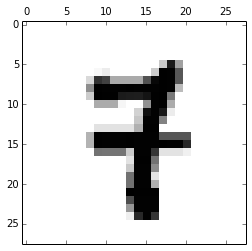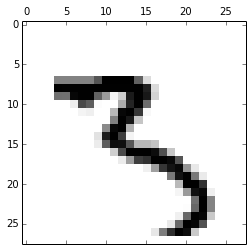# Engineered By: Noelle Milton Vega (PRISMALYTICS, LLC).

# Import scikit-learn, numpy, matplotlib and other python3 modules necessary for this Linear Regression project.
import sklearn.datasets
import sklearn.linear_model
import sklearn.utils
import matplotlib.pyplot
import numpy as np


The MNIST dataset is conveniently bundled into the skearn distribution. Since we're implementing Binary (and not Multinomial) Logistic Regression, only two of the ten (digit) classes available in this dataset are needed to train and test our classification model. We arbitrarily selected digit-classes 3 and 7 for this example. To extract samples and corresponding labels for digit-classes 3 and 7 only, we'll employ a trick that creates a boolean numpy selector-array via a conditional expression.

digit_a = 3
digit_b = 7

mnist = sklearn.datasets.fetch_mldata("MNIST original") # A dict-like object with the following attributes:
# mnist.data   : A numpy array containing the dataset samples.      Shape: (70000, 784). dtype: uint8
# mnist.target : A numpy array containing the corresponding labels. Shape: (70000,). dtype: float64

samples = np.vstack([mnist.data[mnist.target == digit_a],  # Select all samples that are labeled 3.
mnist.data[mnist.target == digit_b]]) # Select all samples that are labeled 7.

# And next we extract labels that 'positionally' correspond
# to the samples selected above. We also convert to uint8 dtype,
labels = np.hstack([mnist.target[mnist.target == digit_a], # which is sufficient and helpful later on.
mnist.target[mnist.target == digit_b]]).astype(numpy.uint8, copy=False)

# The above created subset samples & labels by sequentially stacking 'digit_a' entries followed by 'digit_b' entries.
# We therefore need to shuffle the deck (so to speak) so that when we partition the data into Training and Testing sets,
# each will consist of a roughly 50/50 mix of each binary class. Note: resample() is the same as shuffle()
(samples, labels) = sklearn.utils.shuffle(samples, labels)


Next, we should confirm that the subset samples and corresponding subset labels have the expected shape, dimension, etc.

print('Number of \'samples\' in the extracted dataset (samples.shape):', samples.shape)
print('Number of \'labels\' in extracted dataset (labels.shape):', labels.shape)
print('Number of \'features\' in each sample (samples.shape):', samples.shape)
print('Distinct classes in the \'labels\' vector:', repr(np.unique(labels)))

Number of 'samples' in the extracted dataset (samples.shape): 14434
Number of 'labels' in extracted dataset (labels.shape): 14434
Number of 'features' in each sample (samples.shape): 784
Distinct classes in the 'labels' vector: array([3, 7], dtype=uint8)



As mentioned, each sample comes in the form of a vector of length 784. Consecutive groups of 28 values in the vector represent one row (beginning with row-1) of the 28-pixels-wide by 28-pixels-high handwritten digit that it represents. Knowing this allows us plot it (a luxury rarely avilable in higher dimensional problems). We randomly select one of the extracted samples and plot it's vector here to illustrate what the handwritted digit it represents looks like.

idx = np.random.randint(0, samples.shape)
print("This is an image-plot of a randomly selected vector/sample representing a handwritten digit: %d" % (labels[idx]))
matplotlib.pyplot.rc("image", cmap="binary")
matplotlib.pyplot.matshow(samples[idx].reshape(28, 28))

This is an image-plot of a randomly selected vector/sample representing a handwritten digit: 7Next we divide the dataset into two mutually-exlusive & collectively exhaustive subsets: One to Train with and the other to Test with. We'll stick to simple fractional splitting of a (re)shuffed collection to create both sets here, though this is where you might otherwise consider applying some form of LOO-XVE technique for enhanced cross-validation of trained-models. After splitting, we'll finally check that each set is comprised of roughly 50% 3s-digits (Class-A), and roughly 50% 7s-digits (Class-B).

(samples, labels) = sklearn.utils.shuffle(samples, labels) # We don't cross-validate here, so at least re-shuffle each time.
train_qty = int(samples.shape * 0.85) # We'll use 85%/15% split for Training/Testing.
#
training_samples = samples[0:train_qty,:]
training_labels = labels[0:train_qty]
testing_samples = samples[train_qty:,:]
testing_labels = labels[train_qty:]
#
bincounts_training = np.bincount(training_labels) # See doc for 'scipy.stats.itemfreq()' as an alternative.
bincounts_testing  = np.bincount(testing_labels)
print('Quantity of Class-A/Class-B items in training set: %d / %d' % (bincounts_training[digit_a], bincounts_training[digit_b]))
print('Quantity of Class-A/Class-B items in testing set:  %d / %d' % (bincounts_testing[digit_a], bincounts_testing[digit_b]))

Quantity of Class-A/Class-B items in training set: 6105 / 6163
Quantity of Class-A/Class-B items in testing set:  1036 / 1130



We are finally ready to train a Logistic Regression/Classification model by feeding it our training samples, along with the classes (labels) to which they belong.

clf = sklearn.linear_model.LogisticRegression()
clf.fit(training_samples, training_labels)

LogisticRegression(C=1.0, class_weight=None, dual=False, fit_intercept=True,
intercept_scaling=1, penalty='l2', random_state=None, tol=0.0001)


Now, let's evaluate the accuracy score of our trained model using the Training-set and then the Test-set. We expect 100% accuracy with the Training-set because that is what the model was trained with. And we would like Test-set driven accuracy scores to be as close to 100% as possible; though how well the latter is achieved depends on the model used, the input parameters, the training data, and many times the 'art' supplied by the data scientist.

print('Accuracy for training set: %.2f%%' % (clf.score(training_samples, training_labels) * 100)) # Should always be 100%
print('Accuracy for testing set: %.2f%%' % (clf.score(testing_samples, testing_labels) * 100))    # As close to 100% desired.

Accuracy for training set: 100.00%
Accuracy for testing set: 98.48%



The accuracy score using the Test-set is less than 100%. This is normal and expected. Still, let's get the indices of the test samples for which the PREDICTED LABEL differed from the known TRUE LABEL. This will show how many mis-classifications (incorrect predictions) there were.

misclassified_indices = (clf.predict(testing_samples) != testing_labels).nonzero() # Returns a tuple, so  is needed.
print('The number of incorrect/mis-classified Test sample predictions is:', misclassified_indices.size)
print('The index location of these incorrect/mis-classified Test samples are:\n\t', misclassified_indices)

The number of incorrect/mis-classified Test sample predictions is: 33
The index location of these incorrect/mis-classified Test samples are:
[   8   26   63  138  390  456  515  539  592  624  663  811  873  878  921
972 1105 1123 1230 1245 1250 1258 1419 1437 1441 1455 1487 1520 1809 1812
1851 2129 2139]



Finally, let's randomly select one of the samples whose prediction/classification was incorrect, and plot it to see if we can visualize why the predictor got it wrong; meaning, why it predicted a 3 digit when it was actually a 7 digit, or vice versa.

a_misclassified_index = misclassified_indices[np.random.randint(0, misclassified_indices.size, 1)]

print('True -vs- Predicted classification for mis-classified test sample at index number %i: [True: %i / Pred: %i]' % \
(a_misclassified_index,
testing_labels[a_misclassified_index],
clf.predict(testing_samples[a_misclassified_index])))

print('We plot the image here to see if there are visual clues as to why mis-classification occurred:\n')
plt.matshow(testing_samples[a_misclassified_index].reshape(28, 28))

True -vs- Predicted classification for mis-classified test sample at index number 1441: [True: 3 / Pred: 7]

We plot the image here to see if there are visual clues as to why mis-classification occurred. Indeed, here we see that the
vertically stretched 3 has some resemblance to a 'dashed' number 7 (plotted above). Had there been more similarly written number 3 samples, perhaps the classifier would learn to predict 3 instead.